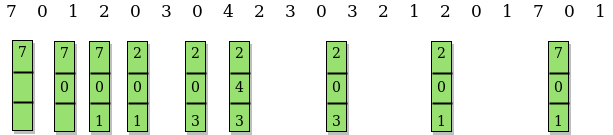# Optimal Page Replacement Algorithm

Prerequisite: Page Replacement Algorithms

In operating systems, whenever a new page is referred and not present in memory, page fault occurs and Operating System replaces one of the existing pages with newly needed page. Different page replacement algorithms suggest different ways to decide which page to replace. The target for all algorithms is to reduce number of page faults.
In this algorithm, OS replaces the page that will not be used for the longest period of time in future.

Examples :

```Input : Number of frames, fn = 3
Reference String, pg[] = {7, 0, 1, 2,
0, 3, 0, 4, 2, 3, 0, 3, 2, 1,
2, 0, 1, 7, 0, 1};
Output : No. of hits = 11
No. of misses = 9

Input : Number of frames, fn = 4
Reference String, pg[] = {7, 0, 1, 2,
0, 3, 0, 4, 2, 3, 0, 3, 2};
Output : No. of hits = 7
No. of misses = 6
```

## Recommended: Please try your approach on {IDE} first, before moving on to the solution.

The idea is simple, for every reference we do following :

1. If referred page is already present, increment hit count.
2. If not present, find if a page that is never referenced in future. If such a page exists, replace this page with new page. If no such page exists, find a page that is referenced farthest in future. Replace this page with new page.`// CPP program to demonstrate optimal page ` `// replacement algorithm. ` `#include ` `using` `namespace` `std; ` ` `  `// Function to check whether a page exists ` `// in a frame or not ` `bool` `search(``int` `key, vector<``int``>& fr) ` `{ ` `    ``for` `(``int` `i = 0; i < fr.size(); i++) ` `        ``if` `(fr[i] == key) ` `            ``return` `true``; ` `    ``return` `false``; ` `} ` ` `  `// Function to find the frame that will not be used ` `// recently in future after given index in pg[0..pn-1] ` `int` `predict(``int` `pg[], vector<``int``>& fr, ``int` `pn, ``int` `index) ` `{ ` `    ``// Store the index of pages which are going ` `    ``// to be used recently in future ` `    ``int` `res = -1, farthest = index; ` `    ``for` `(``int` `i = 0; i < fr.size(); i++) { ` `        ``int` `j; ` `        ``for` `(j = index; j < pn; j++) { ` `            ``if` `(fr[i] == pg[j]) { ` `                ``if` `(j > farthest) { ` `                    ``farthest = j; ` `                    ``res = i; ` `                ``} ` `                ``break``; ` `            ``} ` `        ``} ` ` `  `        ``// If a page is never referenced in future, ` `        ``// return it. ` `        ``if` `(j == pn) ` `            ``return` `i; ` `    ``} ` ` `  `    ``// If all of the frames were not in future, ` `    ``// return any of them, we return 0. Otherwise ` `    ``// we return res. ` `    ``return` `(res == -1) ? 0 : res; ` `} ` ` `  `void` `optimalPage(``int` `pg[], ``int` `pn, ``int` `fn) ` `{ ` `    ``// Create an array for given number of ` `    ``// frames and initialize it as empty. ` `    ``vector<``int``> fr; ` ` `  `    ``// Traverse through page reference array ` `    ``// and check for miss and hit. ` `    ``int` `hit = 0; ` `    ``for` `(``int` `i = 0; i < pn; i++) { ` ` `  `        ``// Page found in a frame : HIT ` `        ``if` `(search(pg[i], fr)) { ` `            ``hit++; ` `            ``continue``; ` `        ``} ` ` `  `        ``// Page not found in a frame : MISS ` ` `  `        ``// If there is space available in frames. ` `        ``if` `(fr.size() < fn) ` `            ``fr.push_back(pg[i]); ` ` `  `        ``// Find the page to be replaced. ` `        ``else` `{ ` `            ``int` `j = predict(pg, fr, pn, i + 1); ` `            ``fr[j] = pg[i]; ` `        ``} ` `    ``} ` `    ``cout << ``"No. of hits = "` `<< hit << endl; ` `    ``cout << ``"No. of misses = "` `<< pn - hit << endl; ` `} ` ` `  `// Driver Function ` `int` `main() ` `{ ` `    ``int` `pg[] = { 7, 0, 1, 2, 0, 3, 0, 4, 2, 3, 0, 3, 2 }; ` `    ``int` `pn = ``sizeof``(pg) / ``sizeof``(pg); ` `    ``int` `fn = 4; ` `    ``optimalPage(pg, pn, fn); ` `    ``return` `0; ` `} `

Output:

```No. of hits = 7
No. of misses = 6```
• The above implementation can optimized using hashing. We can use an unordered_set in place of vector so that search operation can be done in O(1) time.
• Note that optimal page replacement algorithm is not practical as we cannot predict future. However it is used as a reference for other page replacement algorithms.

Attention reader! Don’t stop learning now. Get hold of all the important DSA concepts with the DSA Self Paced Course at a student-friendly price and become industry ready.

My Personal Notes arrow_drop_upCheck out this Author's contributed articles.

If you like GeeksforGeeks and would like to contribute, you can also write an article using contribute.geeksforgeeks.org or mail your article to contribute@geeksforgeeks.org. See your article appearing on the GeeksforGeeks main page and help other Geeks.

Please Improve this article if you find anything incorrect by clicking on the "Improve Article" button below.